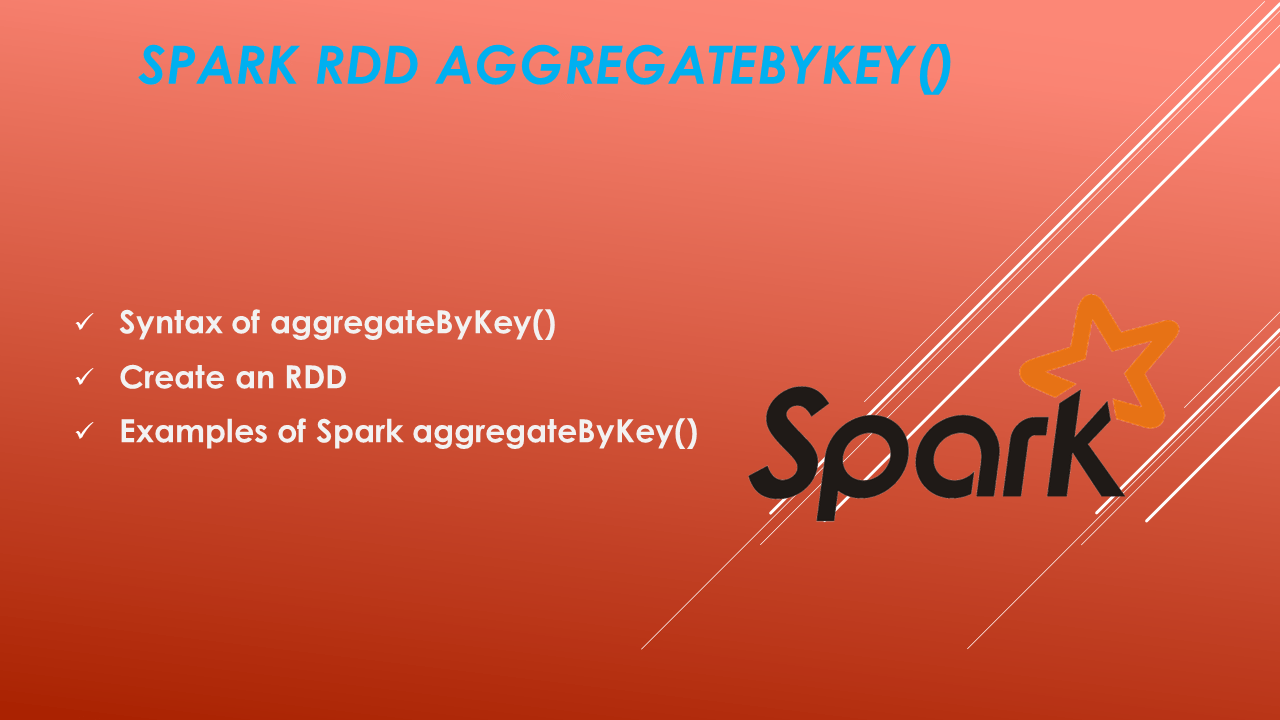# Spark RDD aggregateByKey()

In Spark/Pyspark aggregateByKey() is one of the fundamental transformations of RDD. The most common problem while working with key-value pairs is grouping values and aggregating them considering a standard key. And Spark aggregateByKey() transformation effectively addresses this problem. In this article, we will learn what is aggregateByKey() and how to implement transformation when an aggregation operation is involved.

## 1. Introduction

• Spark RDD aggregateByKey() is one of the aggregate functions (Others are reduceByKey & groupByKey) for aggregating the values of each key, using given combined functions and a neutral “zero value.” and returns a different type of value for that key.
• Performance-wise aggregateByKey is an optimized and broader transformation.
• We should use aggregateByKey when aggregation is required, plus the input and output RDDs are different.
• This is the only aggregation function that allows multiple types of aggregation(Maximum, minimum, average, sum & count) at the same time

## 2. Syntax of aggregateByKey()

Following is the syntax of the RDD aggregateByKey() function.

``````
//Syntax of RDD aggregateByKey()
RDD.aggregateByKey(init_value)(combinerFunc,reduceFunc)
``````

## 2.1 Parameters

1. Initial Value: Initial value (mostly Zero (0)) which will not affect the aggregate values to be collected. For example, 0 would be the initial value to perform sum or count or to perform an operation on String then the initial value will be the empty string.
2. Combiner function: This function accepts two parameters. The second parameter is merged into the first parameter. This function combines/merges values within a single partition.
3. Reduce/Merge function: This function also accepts two parameters. Here parameters are merged into one across RDD partitions.

## 3. Create an RDD

Let us create a test RDD with a tuple of Car Manufacture, Models, and Sales in that year.

``````
// Basic aggregateByKey example in scala
// Creating PairRDD carsRDD with key value pairs,
// Number partitions is 3 defined in parallelize method.
val carsRDD = sc.parallelize(Array(
("Hyundai", "Verna", 2000), ("Hyundai", "Creta", 3000), ("Hyundai", "i20", 9100), ("Hyundai", "i10", 8200),
("Hyundai", "Santro", 6900), ("Maruthi", "Swift", 62000), ("Maruthi", "Baleno", 9007), ("Maruthi", "Breeza", 80000),
("Maruthi", "Ertiga", 70018), ("Tata", "Nexon", 71903), ("Tata", "Safari", 16008), ("Tata", "Tiago", 80347)), 3)
carsRDD.collect()
``````

Yields below output.

``````
//Output:
res0: Array[Any] = Array((Hyundai,Verna,2000), (Hyundai,Creta,3000), (Hyundai,i20,9100), (Hyundai,i10,8200), (Hyundai,Santro,6900), (Maruthi,Swift,62000), (Maruthi,Baleno,9007), (Maruthi,Breeza,80000), (Maruthi,Ertiga,70018), (Tata,Nexon,71903), (Tata,Safari,16008), (Tata,Tiago,80347), 3)
``````

## 4. Examples of Spark aggregateByKey()

Let’s use the above created Spark RDD and run some examples using aggregateByKey().

### 4.1. Get the Maximum sales of a car among all manufacturers.

In this use case, Let’s find the maximum sales by a manufacturer. And we know aggregateByKey has three components zero-value, SeqOperator, and a combiner. The syntax will be like this aggregateByKey(zeroVal)(seqOp, CombOp).

Here the map() function creates a pair RDD by “Manufacture” as “key” and “(Model, Sales)” as “value.” First, SeqOp in each partition fetches the max sales of each Model, and later, the Combiner operation fetches the max Sales of each model by comparing the values for each key in multiple partitions.

``````
//Defining Seqencial Operation and Combiner Operations
//Sequence operation : Finding Maximum Sales from a single partition
def seqOp = (accumulator: Int, element: (String, Int)) =>
if(accumulator > element._2) accumulator else element._2

//Combiner Operation : Finding Maximum Sales out Partition-Wise Accumulators
def combOp = (accumulator1: Int, accumulator2: Int) =>
if(accumulator1 > accumulator2) accumulator1 else accumulator2

//Zero Value: Zero value in our case will be 0 as we are finding Maximum Sales
val zeroVal = 0
val aggrRDD = carsRDD.map(t => (t._1, (t._2, t._3))).aggregateByKey(zeroVal)(seqOp, combOp)

# Print result
aggrRDD.collect foreach println
``````

Yields below output.

``````
//Output:
(Tata,80347)
(Hyundai,9100)
(Maruthi,80000)
``````

### 4.2. Get Maximum Sales of Cars for a Manufacture

In this use case, we try to find the car and maximum sales scored by the manufacturer. And we know aggregateByKey has three components zero-value, Seqoperator, and combiner. The syntax will be like this aggregateByKey(zeroVal)(seqOp, CombOp).

Here the map() function creates a pair RDD by “Manufacture” as “key” and “(Model, Sales)” as “value.” First, SeqOp in each partition fetches the max sales of each Model, and later, the Combiner operation fetches the max Sales of each model by comparing the values for each key in multiple partitions.

``````
// Let's Print Subject name along with Maximum Marks
println("student name,Subject along with Maximum Marks")

// Defining Seqencial Operation and Combiner Operations
def seqOp = (accumulator: (String, Int), element: (String, Int)) =>
if(accumulator._2 > element._2) accumulator else element

def combOp = (accumulator1: (String, Int), accumulator2: (String, Int)) =>
if(accumulator1._2 > accumulator2._2) accumulator1 else accumulator2

// Zero Value: Zero value in our case will be tuple with blank subject name and 0
val zeroVal = ("", 0)
val aggrRDD = carsRDD.map(t => (t._1, (t._2, t._3)))
.aggregateByKey(zeroVal)(seqOp, combOp)

# Print
aggrRDD.collect foreach println
``````

Yields below output.

``````
//Output:
(Tata,(Tiago,80347))
(Hyundai,(i20,9100))
(Maruthi,(Breeza,80000))
``````

## 5. Conclusion

In this article, you have learned bout Spark/Pyspark RDD aggregationByKey() which could be a better alternative to groupByKey transformation when an aggregation operation is involved. And we used this to calculate the maximum operation of sales achieved by car manufacturers.

## Related Articles

### rimmalapudi

Data Engineer. I write about BigData Architecture, tools and techniques that are used to build Bigdata pipelines and other generic blogs.